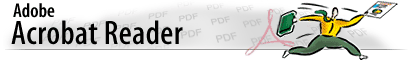Mathematical Sciences 403

# Introduction to Statistical Theory

## Course Objective

### This course is designed to introduce the student to mathematical statistics and statistical inference. It is designed to put to use some of the concepts in probability (MthSc 400) to make statistical inference that can arise in real world applications. Although there will be some review of some of these concepts, it is assumed and expected that there is sufficient knowledge of probability. With respect to statistical inference, we will discuss those foundational issues imperative to the practical application of statistics. Being that the first four chapters of the text addresses non-inferential data analysis, probability, and probability distributions, we will briefly review a couple of topics from these chapters discussed in lecture notes and begin in earnest with chapter 5, and cover chapters 5, 6, 7, 8, 10.1,11 omitting 11.5 and 12.1,12.3-12.4. Topics covered will include: sampling distributions, point and interval estimation, maximum likelihood estimators, method of moments, least squares estimators, tests of hypotheses, likelihood ratio methods, regression and correlation analysis, and introduction to analysis of variance. There will be several statistical and mathematical packages used to simulate distributions and probabilitic processes that lead to statistical applications. Students should be familiar with one or more of the following: Matlab, SAS, and/or Statistix.## Current Enrollment:• Enrollees## First Day's Handouts Syllabus & Review Notes• Course Syllabus
• Probability Distributions
• Moment Generating Functions## Class Notes....Updated and Corrected Frequently## Assignments, Due Dates and Solutions to Previous Assignments (in Adobe Acrobat pdfs)

• MthSc403 Students Assignments:
• MthSc 403H/MthSc 603 Students Assignment:• Get Your Acrobat Reader Here!